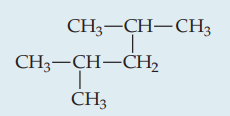×
Get Full Access to Chemistry: The Central Science - 12 Edition - Chapter 24 - Problem 1pe
Get Full Access to Chemistry: The Central Science - 12 Edition - Chapter 24 - Problem 1pe

×

# Naming AlkanesGive the systematic name for the followingISBN: 9780321696724 27

## Solution for problem 1PE Chapter 24

Chemistry: The Central Science | 12th Edition

• Textbook Solutions
• 2901 Step-by-step solutions solved by professors and subject experts
• Get 24/7 help from StudySoup virtual teaching assistantsChemistry: The Central Science | 12th Edition

4 5 1 269 Reviews
21
4
Problem 1PE

Name the following alkane:Step-by-Step Solution:
Step 1 of 3

Step 2 of 3

Step 3 of 3

##### ISBN: 9780321696724

Since the solution to 1PE from 24 chapter was answered, more than 277 students have viewed the full step-by-step answer. This full solution covers the following key subjects: alkane, name, give, Alkanes, naming. This expansive textbook survival guide covers 49 chapters, and 5471 solutions. Chemistry: The Central Science was written by and is associated to the ISBN: 9780321696724. The full step-by-step solution to problem: 1PE from chapter: 24 was answered by , our top Chemistry solution expert on 04/03/17, 07:58AM. The answer to “?Name the following alkane:” is broken down into a number of easy to follow steps, and 4 words. This textbook survival guide was created for the textbook: Chemistry: The Central Science, edition: 12.

## Discover and learn what students are asking

Calculus: Early Transcendental Functions : Basic Differentiation Rules and Rates of Change
?Finding a Value In Exercises 65–70, find k such that the line is tangent to the graph of the function. Function

Calculus: Early Transcendental Functions : Integration by Tables and Other Integration Techniques
?In Exercises 47-52, verify the integration formula. \(\int \frac{u^{n}}{\sqrt{a+b u}} d u=\frac{2}{(2 n+1) b}\left(u^{n} \sqrt{a+b u}-n a \in

Statistics: Informed Decisions Using Data : Introduction to the Practice of Statistics
?A(n)_________ is a person or object that is a member of the population being studied.

Unlock Textbook Solution